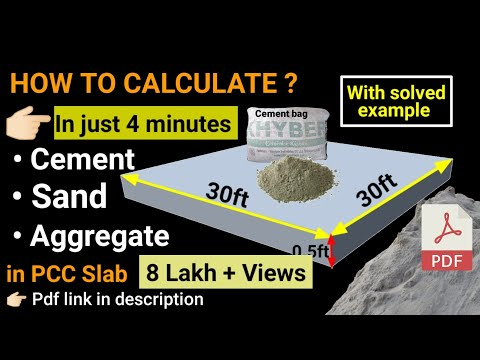# How To Calculate The Amount Of ConcreteVideo: How to calculate cement sand and aggregate quantity in concrete | material quantity calculation | 2023, February

An accurate calculation of the amount of concrete to be placed in the formwork is one of the conditions for the competent performance of construction work. Excess concrete, as a rule, has nowhere to use. The mixer will either take the ordered and prepaid concrete, or dump it to the specified place, creating a "monument" to your bungling. Violation of the construction technology due to the lack of concrete will lead to even more serious consequences. Consider, using the example of a strip foundation, how to avoid such mistakes.

## Necessary

• - ruler,
• - roulette,
• - calculator.

## Instructions

### Step 1

Commodity concrete of various grades, which is a semi-liquid mass, depending on its composition, has an unequal shrinkage - the less cement and water it contains, the less changes in the volume of concrete during hardening. In addition, other factors also affect the amount of concrete shrinkage, for example, the method of its compaction: when bayonetting with a shovel, shrinkage is less than when compacting concrete with a deep vibrator. When calculating the required amount of concrete, a single average statistical shrinkage coefficient is usually used, equal to 1.015-1.02.

### Step 2

Calculate the preliminary volume of the concrete structure, according to the project (sketch). To do this, you need to multiply the width, height and length of the foundation.

### Step 3

After the construction of the formwork, check that the height, width and length of the foundation correspond to the design values. Small deviations in the dimensions in a large direction lead to a significant increase in the required amount of concrete. The use of inventory formwork made of steel frames and moisture-resistant plywood makes it possible to minimize these errors.

### Step 4

Multiply the resulting volume by the shrinkage factor.

### Step 5

Subtract the volume of all openings and niches for utility networks (pipes, ducts) from the obtained value. To do this, you need to calculate the corresponding volumes using the formulas for determining the volume of a cylinder (area of ​​a circle multiply the height) or a parallelepiped (length multiplied by width and height). The resulting value will be the amount of concrete that is needed to fill this structure.